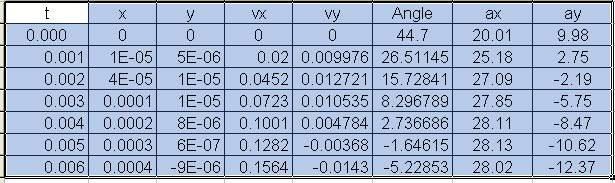# Interceptor rocket?

## Homework Statement

A 5000kg interceptor rocket is launched at an angle of 44.7 degrees. The thrust of the rocket motor is 140,700N
a) Find an equation y(x) that describes the rocket's trajectory
b) What shape is the trajectory?
c) At what elevation does the rocket reach the speed of sound, 330m/s?

## Homework Equations

kinematic equations
Newton's laws

## The Attempt at a Solution

The problem sheet hint tells me that the angle is not 44.7 degrees. So is it right that it should be 45.3 degrees then? Furthermore, speed initial is 0m/s correct?

Delphi51
Homework Helper
The angle will vary. The force of gravity will reduce the angle. It will be a curved trajectory with the angle continuously changing. Yes, it appears that the initial velocity is zero.

Write the equations for vertical and horizontal motion to find out what happens. Looks interesting!

The angle will vary. The force of gravity will reduce the angle. It will be a curved trajectory with the angle continuously changing. Yes, it appears that the initial velocity is zero.

Write the equations for vertical and horizontal motion to find out what happens. Looks interesting!

I don't see how to start off a then because I get something over the initial velocity squared, which is zero. As a result, it should not be defined. Any hints?

Delphi51
Homework Helper
Oops, sorry, I shouldn't have commented. I don't know how to solve it. Quite a little set of differential equations involved! I can find the answers using a computer simulation but I've lost the ability to do varying acceleration problems via calculus.

Funny, according to my little spreadsheet model, it doesn't get far off the ground.
The component of the thrust in the vertical direction is very soon overcome by gravity and the rocket loses angle very quickly.Last edited: